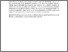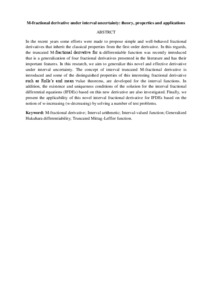# M-fractional derivative under interval uncertainty: theory, properties and applications

## Citation

S., Salahshour and A., Ahmadian and S., Abbasbandy and D., Baleanu (2018) M-fractional derivative under interval uncertainty: theory, properties and applications. Chaos, Solitons and Fractals, 117. 84 - 93. ISSN 0960-0779

## Abstract

In the recent years some efforts were made to propose simple and well-behaved fractional derivatives that inherit the classical properties from the first order derivative. In this regards, the truncated M-fractional derivative for α-differentiable function was recently introduced that is a generalization of four fractional derivatives presented in the literature and has their important features. In this research, we aim to generalize this novel and effective derivative under interval uncertainty. The concept of interval truncated M-fractional derivative is introduced and some of the distinguished properties of this interesting fractional derivative such as Rolle’s and mean value theorems, are developed for the interval functions. In addition, the existence and uniqueness conditions of the solution for the interval fractional differential equations (IFDEs) based on this new derivative are also investigated. Finally, we present the applicability of this novel interval fractional derivative for IFDEs based on the notion of w-increasing (w-decreasing) by solving a number of test problems.Preview
Text
M-fractional derivative.pdfView Item